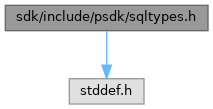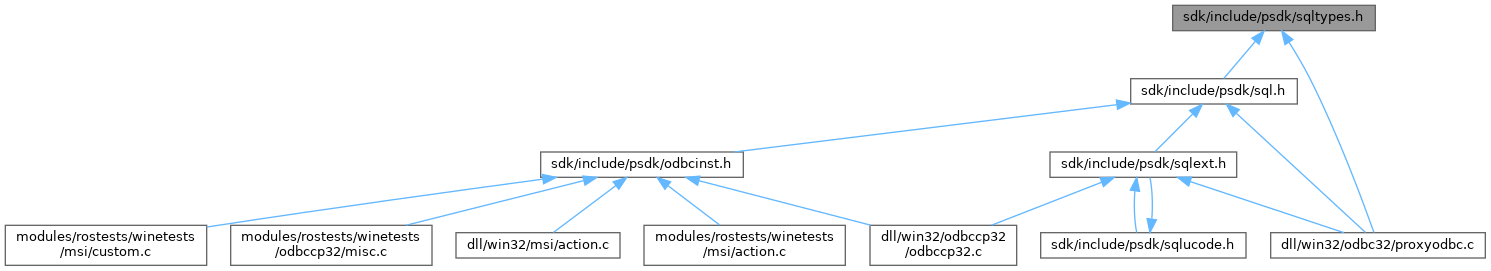ReactOS  0.4.15-dev-3728-ga92304f
sqltypes.h File Reference
#include <stddef.h>
Include dependency graph for sqltypes.h:This graph shows which files directly or indirectly include this file:Go to the source code of this file.

Classes

struct  tagDATE_STRUCT

struct  tagTIME_STRUCT

struct  tagTIMESTAMP_STRUCT

Macros

#define SQL_API   __stdcall

#define __need_wchar_t

#define SQLLEN   SQLINTEGER

#define SQLULEN   SQLUINTEGER

#define SQLSETPOSIROW   SQLUSMALLINT

Typedefs

typedef signed char SCHAR

typedef long SDWORD

typedef short SWORD

typedef ULONG UDWORD

typedef USHORT UWORD

typedef signed long SLONG

typedef signed short SSHORT

typedef double SDOUBLE

typedef double LDOUBLE

typedef float SFLOAT

typedef PVOID PTR

typedef PVOID HENV

typedef PVOID HDBC

typedef PVOID HSTMT

typedef short RETCODE

typedef UCHAR SQLCHAR

typedef SDWORD SQLINTEGER

typedef SWORD SQLSMALLINT

typedef UDWORD SQLUINTEGER

typedef UWORD SQLUSMALLINT

typedef PVOID SQLPOINTER

typedef voidSQLHENV

typedef voidSQLHDBC

typedef voidSQLHSTMT

typedef SQLSMALLINT SQLRETURN

typedef HWND SQLHWND

typedef SQLULEN BOOKMARK

typedef SQLLEN SQLROWOFFSET

typedef SQLULEN SQLROWCOUNT

typedef SQLULEN SQLTRANSID

typedef wchar_t SQLWCHAR

typedef SQLCHAR SQLTCHAR

typedef struct tagDATE_STRUCT DATE_STRUCT

typedef struct tagTIME_STRUCT TIME_STRUCT

typedef struct tagTIMESTAMP_STRUCT TIMESTAMP_STRUCT

◆ __need_wchar_t

 #define __need_wchar_t

Definition at line 11 of file sqltypes.h.

◆ SQL_API

 #define SQL_API   __stdcall

Definition at line 8 of file sqltypes.h.

◆ SQLLEN

 #define SQLLEN   SQLINTEGER

Definition at line 46 of file sqltypes.h.

◆ SQLSETPOSIROW

 #define SQLSETPOSIROW   SQLUSMALLINT

Definition at line 48 of file sqltypes.h.

◆ SQLULEN

 #define SQLULEN   SQLUINTEGER

Definition at line 47 of file sqltypes.h.

◆ BOOKMARK

 typedef SQLULEN BOOKMARK

Definition at line 51 of file sqltypes.h.

◆ DATE_STRUCT

 typedef struct tagDATE_STRUCT DATE_STRUCT

◆ HDBC

 typedef PVOID HDBC

Definition at line 26 of file sqltypes.h.

◆ HENV

 typedef PVOID HENV

Definition at line 25 of file sqltypes.h.

◆ HSTMT

 typedef PVOID HSTMT

Definition at line 27 of file sqltypes.h.

◆ LDOUBLE

 typedef double LDOUBLE

Definition at line 22 of file sqltypes.h.

◆ PTR

 typedef PVOID PTR

Definition at line 24 of file sqltypes.h.

◆ RETCODE

 typedef short RETCODE

Definition at line 28 of file sqltypes.h.

◆ SCHAR

 typedef signed char SCHAR

Definition at line 14 of file sqltypes.h.

◆ SDOUBLE

 typedef double SDOUBLE

Definition at line 21 of file sqltypes.h.

◆ SDWORD

 typedef long SDWORD

Definition at line 15 of file sqltypes.h.

◆ SFLOAT

 typedef float SFLOAT

Definition at line 23 of file sqltypes.h.

◆ SLONG

 typedef signed long SLONG

Definition at line 19 of file sqltypes.h.

◆ SQLCHAR

 typedef UCHAR SQLCHAR

Definition at line 29 of file sqltypes.h.

◆ SQLHDBC

 typedef void* SQLHDBC

Definition at line 36 of file sqltypes.h.

◆ SQLHENV

 typedef void* SQLHENV

Definition at line 35 of file sqltypes.h.

◆ SQLHSTMT

 typedef void* SQLHSTMT

Definition at line 37 of file sqltypes.h.

◆ SQLHWND

 typedef HWND SQLHWND

Definition at line 39 of file sqltypes.h.

◆ SQLINTEGER

 typedef SDWORD SQLINTEGER

Definition at line 30 of file sqltypes.h.

◆ SQLPOINTER

 typedef PVOID SQLPOINTER

Definition at line 34 of file sqltypes.h.

◆ SQLRETURN

 typedef SQLSMALLINT SQLRETURN

Definition at line 38 of file sqltypes.h.

◆ SQLROWCOUNT

 typedef SQLULEN SQLROWCOUNT

Definition at line 53 of file sqltypes.h.

◆ SQLROWOFFSET

 typedef SQLLEN SQLROWOFFSET

Definition at line 52 of file sqltypes.h.

◆ SQLSMALLINT

 typedef SWORD SQLSMALLINT

Definition at line 31 of file sqltypes.h.

◆ SQLTCHAR

 typedef SQLCHAR SQLTCHAR

Definition at line 60 of file sqltypes.h.

◆ SQLTRANSID

 typedef SQLULEN SQLTRANSID

Definition at line 54 of file sqltypes.h.

◆ SQLUINTEGER

 typedef UDWORD SQLUINTEGER

Definition at line 32 of file sqltypes.h.

◆ SQLUSMALLINT

 typedef UWORD SQLUSMALLINT

Definition at line 33 of file sqltypes.h.

◆ SQLWCHAR

 typedef wchar_t SQLWCHAR

Definition at line 56 of file sqltypes.h.

◆ SSHORT

 typedef signed short SSHORT

Definition at line 20 of file sqltypes.h.

◆ SWORD

 typedef short SWORD

Definition at line 16 of file sqltypes.h.

◆ TIME_STRUCT

 typedef struct tagTIME_STRUCT TIME_STRUCT

◆ TIMESTAMP_STRUCT

 typedef struct tagTIMESTAMP_STRUCT TIMESTAMP_STRUCT

◆ UDWORD

 typedef ULONG UDWORD

Definition at line 17 of file sqltypes.h.

◆ UWORD

 typedef USHORT UWORD

Definition at line 18 of file sqltypes.h.